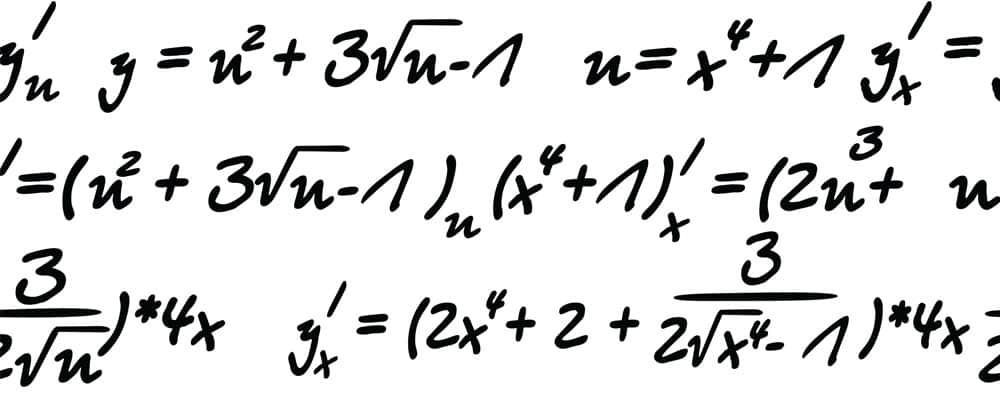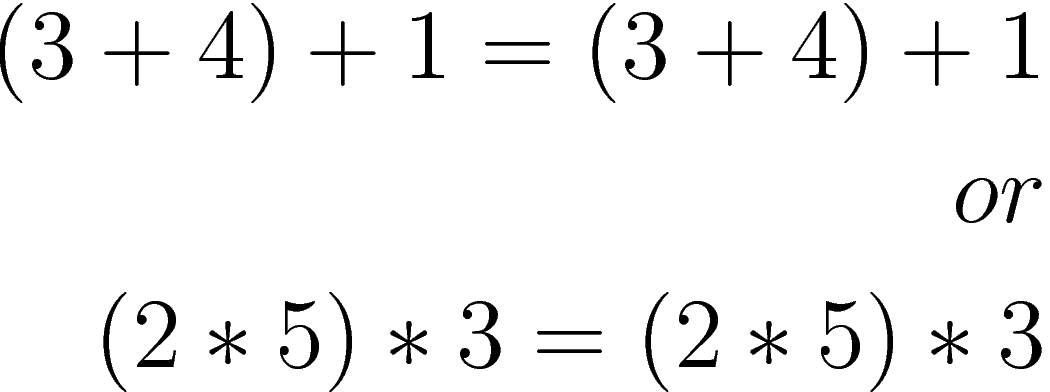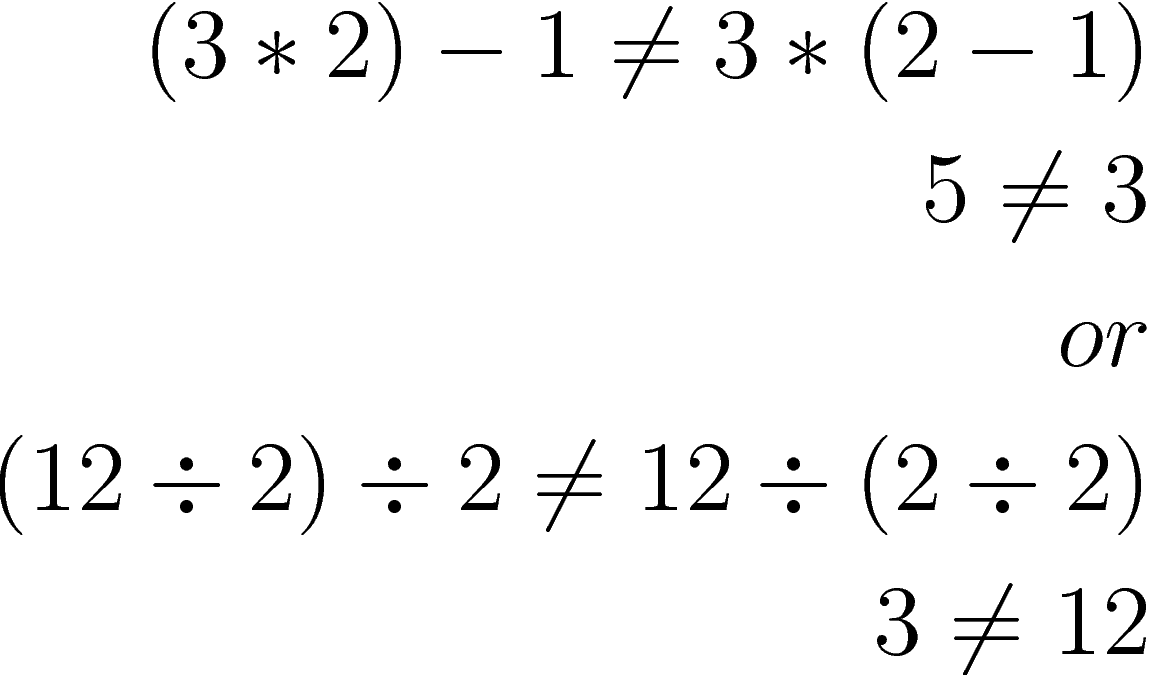# Real Number Properties

In this post, we will examine several properties of math. The word property in this context means characteristic or trait. In other words, we are going to look at characteristics of math.Communicative Property

The communicative property applies both to addition and multiplication. To express this simply the communicative property states that the order the of the numbers does not matter when we add or multiply. Examine the example belowWhether 8 =+ 9 or 9+ 8 it doesn’t matter as the answer is the same. However, this does not work for subtraction or division because the order of the numbers is critical. Consider the followingThe point is order does not matter for addition and multiplication but the order does matter for subtraction and division.

Associative Property

The associative property has to do with the grouping of numbers. Often, numbers are grouped in equations with parentheses or brackets. When this is done for an addition and multiplication problem there is no change to the results. You can see this in the example below.However, the associative property does not work with subtraction and division as the order of the numbers affects the final values as shown below.Identity Property

The identity property of addition states that any number added to zero does not change. The identity property of multiplication states that any number multiplied by 1 does not change.

Inverse Property

There are also inverse properties for addition and nultiplication. The inverse property of addition states that adding the opposite value to a real number will result in zero. Or in other wordsThe inverse property of multiplication states any number multiplied by its reciprocal will equal 1. Or as shown belowDistributive Property

The distributive property is used to get rid of parentheses in order to simplify expressions. This is hard to explain but easy to figure out with an example as shown below.In the example, you can see that we distributed the 6 by multiplying it with the values within the parentheses. By doing this we were able to remove the parentheses.

Conclusion

Understanding these properties are useful when it is necessary to do more complex calculations. When you know how the numbers should behave it is easier to identify when they do not behave appropriately.